# Shazab

17 de Sep de 2016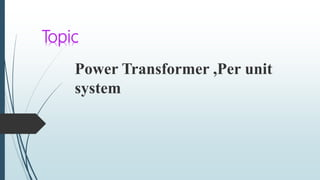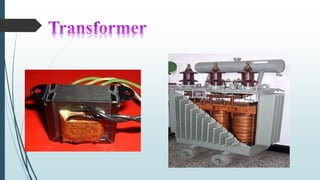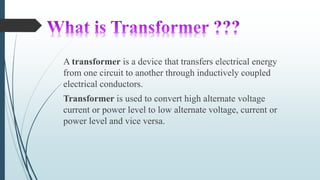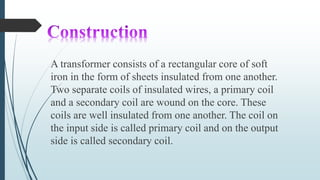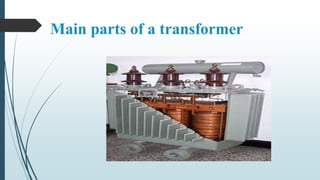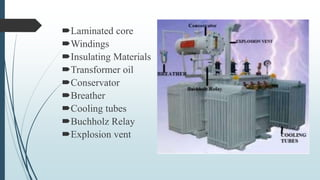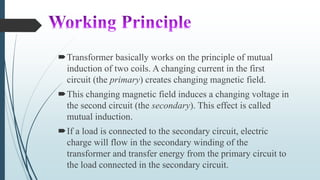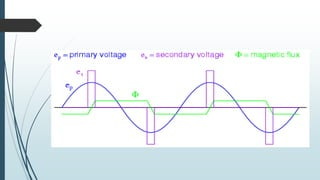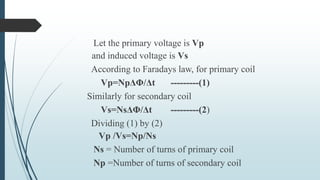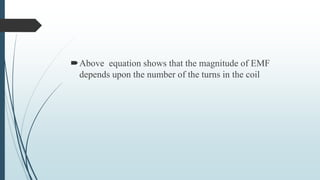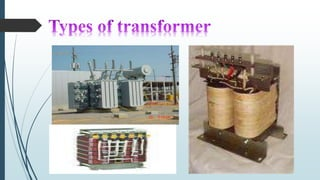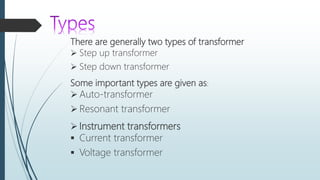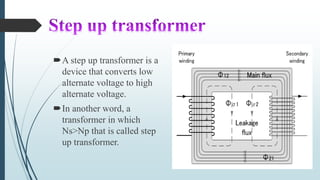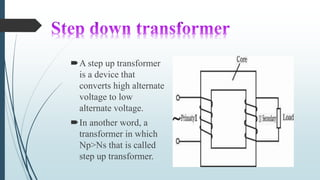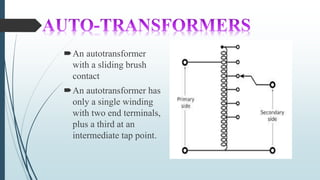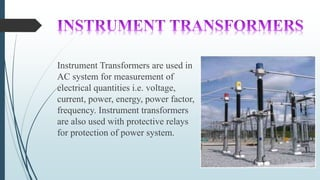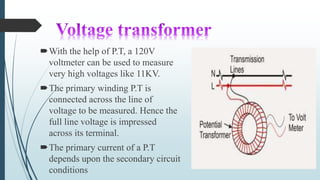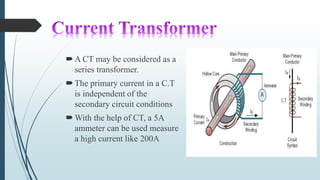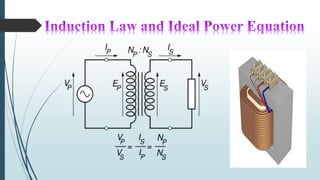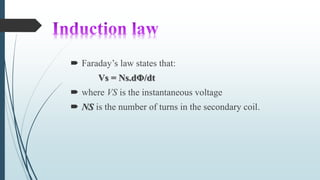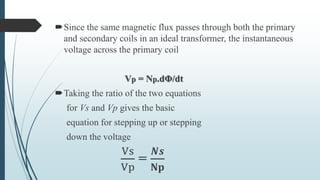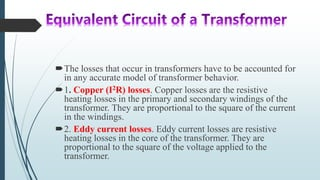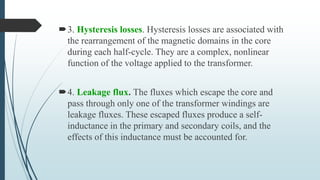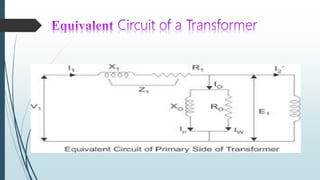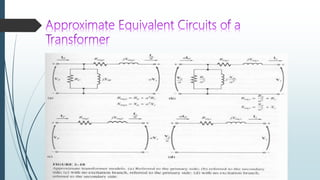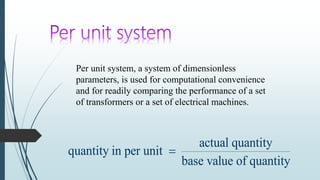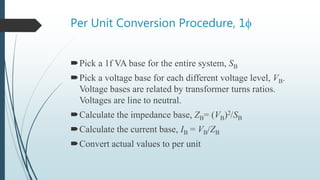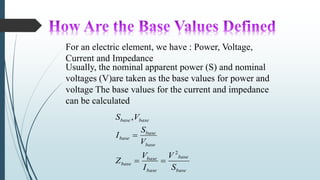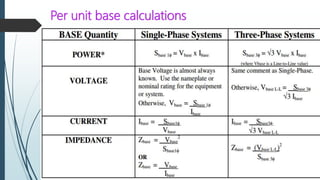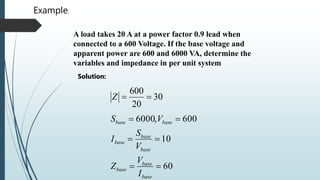1 de 31

### Shazab

• 1. Power Transformer ,Per unit system Topic
• 4. A transformer is a device that transfers electrical energy from one circuit to another through inductively coupled electrical conductors. Transformer is used to convert high alternate voltage current or power level to low alternate voltage, current or power level and vice versa.
• 5. A transformer consists of a rectangular core of soft iron in the form of sheets insulated from one another. Two separate coils of insulated wires, a primary coil and a secondary coil are wound on the core. These coils are well insulated from one another. The coil on the input side is called primary coil and on the output side is called secondary coil.
• 6. Main parts of a transformer
• 7. Laminated core Windings Insulating Materials Transformer oil Conservator Breather Cooling tubes Buchholz Relay Explosion vent
• 8. Transformer basically works on the principle of mutual induction of two coils. A changing current in the first circuit (the primary) creates changing magnetic field. This changing magnetic field induces a changing voltage in the second circuit (the secondary). This effect is called mutual induction. If a load is connected to the secondary circuit, electric charge will flow in the secondary winding of the transformer and transfer energy from the primary circuit to the load connected in the secondary circuit.
• 10. Let the primary voltage is Vp and induced voltage is Vs According to Faradays law, for primary coil Vp=NpΔΦ/Δt ---------(1) Similarly for secondary coil Vs=NsΔΦ/Δt ---------(2) Dividing (1) by (2) Vp /Vs=Np/Ns Ns = Number of turns of primary coil Np =Number of turns of secondary coil
• 11. Above equation shows that the magnitude of EMF depends upon the number of the turns in the coil
• 13. There are generally two types of transformer  Step up transformer  Step down transformer Some important types are given as:  Auto-transformer  Resonant transformer  Instrument transformers  Current transformer  Voltage transformer
• 14. A step up transformer is a device that converts low alternate voltage to high alternate voltage. In another word, a transformer in which Ns>Np that is called step up transformer.
• 15. A step up transformer is a device that converts high alternate voltage to low alternate voltage. In another word, a transformer in which Np>Ns that is called step up transformer.
• 16. An autotransformer with a sliding brush contact An autotransformer has only a single winding with two end terminals, plus a third at an intermediate tap point.
• 17. Instrument Transformers are used in AC system for measurement of electrical quantities i.e. voltage, current, power, energy, power factor, frequency. Instrument transformers are also used with protective relays for protection of power system.
• 18. With the help of P.T, a 120V voltmeter can be used to measure very high voltages like 11KV. The primary winding P.T is connected across the line of voltage to be measured. Hence the full line voltage is impressed across its terminal. The primary current of a P.T depends upon the secondary circuit conditions
• 19. A CT may be considered as a series transformer. The primary current in a C.T is independent of the secondary circuit conditions With the help of CT, a 5A ammeter can be used measure a high current like 200A
• 21.  Faraday’s law states that: Vs = Ns.dΦ/dt  where VS is the instantaneous voltage  NS is the number of turns in the secondary coil.
• 22. Since the same magnetic flux passes through both the primary and secondary coils in an ideal transformer, the instantaneous voltage across the primary coil Vp = Np.dΦ/dt Taking the ratio of the two equations for Vs and Vp gives the basic equation for stepping up or stepping down the voltage Vs Vp = 𝑵𝒔 𝐍𝐩
• 23. The losses that occur in transformers have to be accounted for in any accurate model of transformer behavior. 1. Copper (I2R) losses. Copper losses are the resistive heating losses in the primary and secondary windings of the transformer. They are proportional to the square of the current in the windings. 2. Eddy current losses. Eddy current losses are resistive heating losses in the core of the transformer. They are proportional to the square of the voltage applied to the transformer.
• 24. 3. Hysteresis losses. Hysteresis losses are associated with the rearrangement of the magnetic domains in the core during each half-cycle. They are a complex, nonlinear function of the voltage applied to the transformer. 4. Leakage flux. The fluxes which escape the core and pass through only one of the transformer windings are leakage fluxes. These escaped fluxes produce a self- inductance in the primary and secondary coils, and the effects of this inductance must be accounted for.
• 27. actual quantity quantity in per unit base value of quantity  Per unit system, a system of dimensionless parameters, is used for computational convenience and for readily comparing the performance of a set of transformers or a set of electrical machines.
• 28. Per Unit Conversion Procedure, 1f Pick a 1f VA base for the entire system, SB Pick a voltage base for each different voltage level, VB. Voltage bases are related by transformer turns ratios. Voltages are line to neutral. Calculate the impedance base, ZB= (VB)2/SB Calculate the current base, IB = VB/ZB Convert actual values to per unit
• 29. For an electric element, we have : Power, Voltage, Current and Impedance Usually, the nominal apparent power (S) and nominal voltages (V)are taken as the base values for power and voltage The base values for the current and impedance can be calculated base base base base base base base base basebase S V I V Z V S I VS 2 ,  
• 30. Per unit base calculations
• 31. A load takes 20 A at a power factor 0.9 lead when connected to a 600 Voltage. If the base voltage and apparent power are 600 and 6000 VA, determine the variables and impedance in per unit system 60 10 600,6000    base base base base base base basebase I V Z V S I VS 30 20 600 Z Example: Solution: